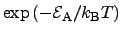## 4.2.6 Simulated Annealing Approach

This optimization follows the observed natural law that thermal annealing (rapid heating and slow cooling) yields more regular crystal structures. This method has been first proposed by METROPOLIS4.9 in 1953  and has been constantly improved [272,273,274,275,276,277,243]. This method is commonly used in semiconductor technology nodes to activate the implanted doping profiles, where the impurities are set to lattice sites of the substrate material. The optimization method uses a similar approach to overcome a trapped situation in local minima. In the hot state, if a local minimum is found, the algorithm searches also in regions different to the local minimizer. This search radius is reduced in each iteration according to the falling temperature, similar to thermal diffusion and BROWNian motion, described by a function proportional to the BOLTZMANN factor. At each iteration of the optimizer, the temperature is decreased like a natural cooling process. Hence, the probability that the algorithm searches in different locations goes asymptotically to zero.

Different implementations of simulated annealing such as adaptive simulated annealing'' [275,276] and very fast simulated annealing''  use different models to described the decreasing temperature. Both implementations use an exponential temperature decay in their models. Advanced versions of the simulated annealing approach also use local optimization techniques and thus optimize the local optima using the exponential temperature decay in the BOLTZMANN factor. Such optimization algorithm start with an initial guess which is locally optimized for instance with a implementation of the LEVENBERG-MARQUARDT optimization algorithm [276,243]. If a local optimum is found the algorithm jumps to a different point with a certain probability determined by a factor proportional to the BOLTZMANN factor and starts another local optimization with the hope of finding a better local optimum as compared to the existing one.

Stefan Holzer 2007-11-19